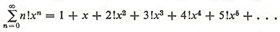A# series

A series is the sum of all or some of the terms of a sequence, which may be finite or infinite. Thus, the series 1 + 4 + 9 + 16 + ... is formed from the sequence 1, 4, 9, 16 ,... A series may or may not converge to a particular value as more and more terms are included. A series is said to be absolutely convergent if the sum of the absolute values of the terms converges; in this case the series converges no matter how the terms of the sum are arranged. Series that are conditionally convergent only converge for some arrangements of the terms and, even then, converge to different values for different arrangements.

A series formed from increasing powers of a variable is a power series; such power series are used to represent many functions.

## Infinite series

An infinite series is an infinite sum of the form a1 + a2 + a3 + ... = (sum from k = 0 to k = infinity) ak. Such series crop up in many areas of modern mathematics. Their development began in the seventeenth century and was continued by Leonhard Euler who, in the process, solved many important problems.

## Power series

A power series is a series of the formThe numbers a1, a2, a3, ... are called the coefficients of the power series. x is a variable.

Polynomials of the form

a0 + a1x + a2x2 + ... + apxp

are also power series with finite numbers of terms.

### Examples of power series

The binomial series, the exponential series, the logarithmic series, the trigonometric series, and the inverse trigonometric series are power series in the variable x.

Every power series possesses an interval of convergence and a radius of convergence. If r is the radius of convergence of a power series, the series converges for all values of x for which |x| < r, while the series diverges for all values of |x| > r. The interval -r < x < +r is referred to as the interval of convergence. The radius of convergence can be 0, in which case the series only converges for the value x = 0. The radius of convergence may also be ∞, in which case the series converges for all values of x.

The exponential seriesconverges for all values of x.

The logarithmic seriesconverges for -1 < x ≤ 1.

The power seriesconverges only for for x = 1.